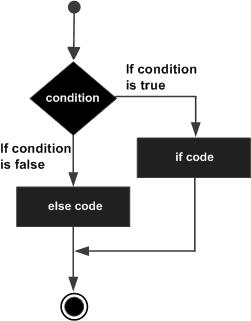# C - if...else statement

An if statement can be followed by an optional else statement, which executes when the Boolean expression is false.

## Syntax

The syntax of an if...else statement in C programming language is −

```if(boolean_expression) {
/* statement(s) will execute if the boolean expression is true */
} else {
/* statement(s) will execute if the boolean expression is false */
}
```

If the Boolean expression evaluates to true, then the if block will be executed, otherwise, the else block will be executed.

C programming language assumes any non-zero and non-null values as true, and if it is either zero or null, then it is assumed as false value.

## Flow Diagram## Example

```#include <stdio.h>

int main () {

/* local variable definition */
int a = 100;

/* check the boolean condition */
if( a < 20 ) {
/* if condition is true then print the following */
printf("a is less than 20\n" );
} else {
/* if condition is false then print the following */
printf("a is not less than 20\n" );
}

printf("value of a is : %d\n", a);

return 0;
}
```

When the above code is compiled and executed, it produces the following result −

```a is not less than 20;
value of a is : 100
```

## If...else if...else Statement

An if statement can be followed by an optional else if...else statement, which is very useful to test various conditions using single if...else if statement.

When using if...else if..else statements, there are few points to keep in mind −

• An if can have zero or one else's and it must come after any else if's.

• An if can have zero to many else if's and they must come before the else.

• Once an else if succeeds, none of the remaining else if's or else's will be tested.

### Syntax

The syntax of an if...else if...else statement in C programming language is −

```if(boolean_expression 1) {
/* Executes when the boolean expression 1 is true */
} else if( boolean_expression 2) {
/* Executes when the boolean expression 2 is true */
} else if( boolean_expression 3) {
/* Executes when the boolean expression 3 is true */
} else {
/* executes when the none of the above condition is true */
}
```

### Example

```#include <stdio.h>

int main () {

/* local variable definition */
int a = 100;

/* check the boolean condition */
if( a == 10 ) {
/* if condition is true then print the following */
printf("Value of a is 10\n" );
} else if( a == 20 ) {
/* if else if condition is true */
printf("Value of a is 20\n" );
} else if( a == 30 ) {
/* if else if condition is true  */
printf("Value of a is 30\n" );
} else {
/* if none of the conditions is true */
printf("None of the values is matching\n" );
}

printf("Exact value of a is: %d\n", a );

return 0;
}
```

When the above code is compiled and executed, it produces the following result −

```None of the values is matching
Exact value of a is: 100
```
c_decision_making.htm